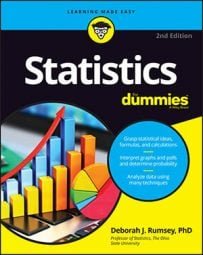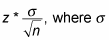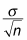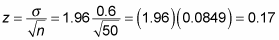##### Statistics For DummiesWhen you need to find a statistical sample mean (or average), you also need to report a margin of error, or MOE, for the sample mean. You can also calculate the margin of error of a sample proportion, which is the amount of "successes" in a sample compared to the whole. The general formula for the margin of error for the sample mean (assuming a certain condition is met — see below) isis the population standard deviation, n is the sample size, and z* is the appropriate z*-value for your desired level of confidence (which you can find in the following table).

Percentage Confidence z*-Value z*-Values for Selected (Percentage) Confidence Levels 80 1.28 90 1.645 95 1.96 98 2.33 99 2.58
Note that these values are taken from the standard normal (Z-) distribution. The area between each z* value and the negative of that z* value is the confidence percentage (approximately). For example, the area between z*=1.28 and z=-1.28 is approximately 0.80. This chart can be expanded to other confidence percentages as well. The chart shows only the confidence percentages most commonly used.

Here are the steps for calculating the margin of error for a sample mean:

1. Find the population standard deviation and the sample size, n.

The population standard deviation, σ, will be given in the problem.

2. Divide the population standard deviation by the square root of the sample size.gives you the standard error.

3. Multiply by the appropriate z*-value (refer to the above table).

For example, the z*-value is 1.96 if you want to be about 95% confident.

The condition you need to meet to use a z*-value in the margin of error formula for a sample mean is either: 1) The original population has a normal distribution to start with, or 2) The sample size is large enough so the normal distribution can be used (that is, the Central Limit Theorem applies).

In general, the sample size, n, should be above about 30 in order for the Central Limit Theorem to be applicable. Now, if it’s 29, don’t panic — 30 is not a magic number, it’s just a general rule of thumb. (The population standard deviation must be known either way.)

Here’s an example: Suppose you’re the manager of an ice cream shop, and you’re training new employees to be able to fill the large-size cones with the proper amount of ice cream (10 ounces each). You want to estimate the average weight of the cones they make over a one-day period, including a margin of error. Instead of weighing every single cone made, you ask each of your new employees to randomly spot check the weights of a random sample of the large cones they make and record those weights on a notepad. For n = 50 cones sampled, the sample mean was found to be 10.3 ounces. Suppose the population standard deviation is 0.6 ounces.

What’s the margin of error? (Assume you want a 95 percent level of confidence.) It’s calculated this way:So to report these results, you say that based on the sample of 50 cones, you estimate that the average weight of all large cones made by the new employees over a one-day period is 10.3 ounces, with a margin of error of plus or minus 0.17 ounces. In other words, the range of likely values for the average weight of all large cones made for the day is estimated (with 95 percent confidence) to be between 10.30 – 0.17 = 10.13 ounces and 10.30 + 0.17 = 10.47 ounces. The new employees appear to be giving out too much ice cream (although you're probably getting great reviews from your customers!).

Notice in this example, the units are ounces, not percentages. When working with and reporting results about data, always remember what the units are. Also, be sure that statistics are reported with their correct units of measure, and if they’re not, ask what the units are.

In cases where n is too small (in general, less than 30) for the Central Limit Theorem to be used, but you still think the data came from a normal distribution, you can use a t*-value instead of a z*-value in your formulas. A t*-value is one that comes from a t-distribution with n – 1 degrees of freedom. In fact, many statisticians go ahead and use t*-values instead of z*-values consistently, because if the sample size is large, t*-values and z*-values are approximately equal anyway.

In addition, for cases where you don’t know the population standard deviation, σ, you can substitute it with s, the sample standard deviation; from there you use a t*-value instead of a z*-value in your formulas as well.